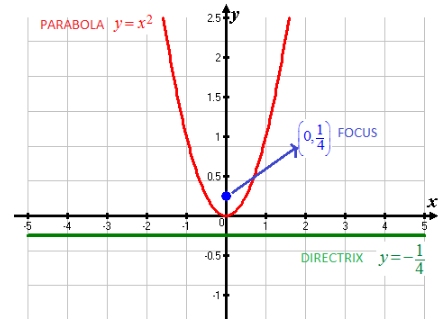# To find the focus and directrix of the parabola y = x 2 and sketch the diagram### Single Variable Calculus: Concepts...

4th Edition
James Stewart
Publisher: Cengage Learning
ISBN: 9781337687805### Single Variable Calculus: Concepts...

4th Edition
James Stewart
Publisher: Cengage Learning
ISBN: 9781337687805

#### Solutions

Chapter B, Problem 48E
To determine

## To calculate: To find the focus and directrix of the parabola y=x2 and sketch the diagram

Expert Solution

The focus of parabola is (0,14) and directrix is y=14

### Explanation of Solution

Given information: Equation of parabola is y=x2

Formula Used:

Parabola is a set of points in the plane that are equidistant from a fixed point F called the focus and a fixed line called the directrix

Calculation:

Equation of parabola is given as

y=x2

Comparing the equation withthe standard equation of parabola x2=4by with focus F(0,b) and directrix y=b

4b=1

On solving,

b=14

Thus,

Focus of parabola is (0,14) and directrix is y=14

On sketching the parabola,Conclusion:

Hence, focus of parabola is (0,14) and directrix is y=14

### Have a homework question?

Subscribe to bartleby learn! Ask subject matter experts 30 homework questions each month. Plus, you’ll have access to millions of step-by-step textbook answers!